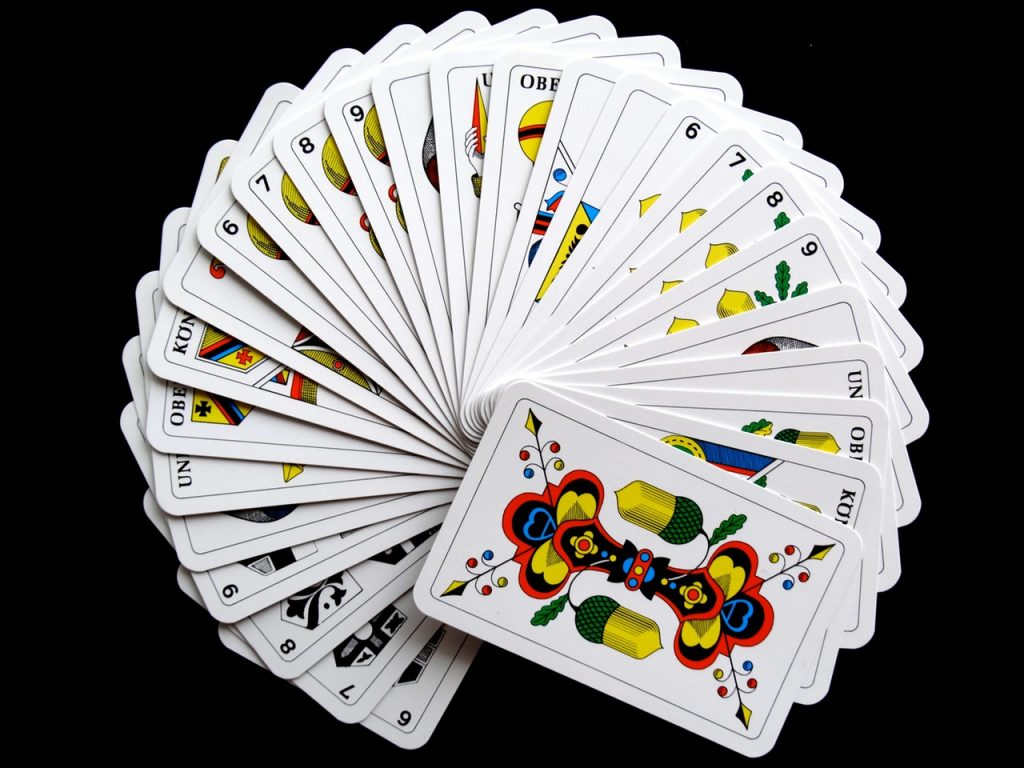June 27, 2022

# What is the probability of getting a heart from 52 cards deck?Here is the detail which you need to have a look on and the capital letters here are used as the representation of an event and the probability of that specified event is donated as P(A).

–    Experiment

An experiment basically is a process in which it yields an observation or a result. There is no compulsion that the experiments would involve the boiling flasks or any colored liquids or the electricity experiments rather they could be simpler relatively. The probability is basically the number of outcomes that would be there in a specified situation and there is an assumption that all the outcomes would be equal so likely.

Simply to know of the probability of the heart in the 52 cards deck requires you to have some simple steps of information about it.

Basically, the standard deck including the playing cards has four suites that are diamonds, spades, hearts, and clubs. Every suite includes thirteen numbers of cards that are ace, king, queen, and jack having 2,3,4,5,6,7,8,9,10 of the ace. Thus the deck as a whole includes 52 cards.

At the time when you want to know the probability of selecting one specific card out of the deck containing 52 cards total, there is an assumption needed to make and that is the cards have been shuffled so well that there are no chances of mistake and another thing that is important to take care of is the visibility of each card in the deck. While the deck has the arrangement of the cards face down, you would never know the value or the suite of the cards.

The solution then is simple as the total number of hearts in a deck is 13 and the cards in a deck are 52 so dividing 13 by 52 gets 0.25 and this is the probability of getting the heart from the deck of cards having 52 of the playing cards.

–   Classical description

The classical definition of probability works well when it is about the determination of the probabilities in the game to check the chances of the outcomes like in the roulette and poker because there is the simple application of the stated description of the probability of the heart card out of 52 as that is simple and defined.

Not using the calculations, there is another one of the best ways which could be used for checking the probability of a certain event which is basically meant as the number of events which you would be familiar with and you will consider that there would be the responses formed for the computation of the specified events to occur in a respectful manner and the frequency of the event to occur. Until there is a calculation of a large number of probabilities for you and while you would get more experience to probabilities, there would be some terminology chances as well for you to have a look on.